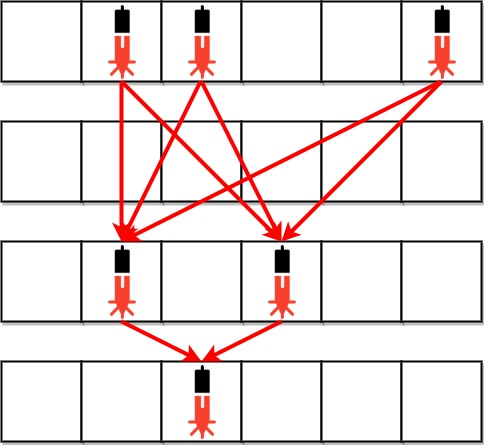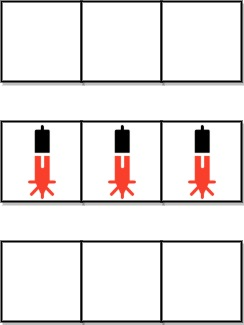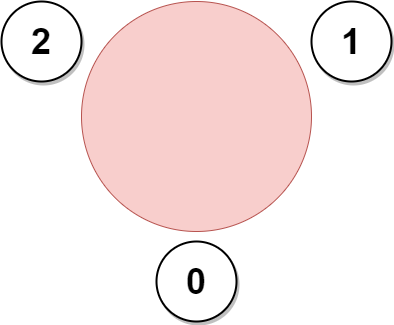# weekly-contest-274

## A

### Statement

``````输入：s = "aaabbb"

'a' 位于下标 0、1 和 2 ；而 'b' 位于下标 3、4 和 5 。

``````

``````输入：s = "abab"

``````

``````输入：s = "bbb"

``````

• `1 <= s.length <= 100`
• `s[i]``'a'``'b'`

Given a string `s` consisting of only the characters `'a'` and `'b'`, return `true` if every `'a'` appears before every `'b'` in the string. Otherwise, return `false`.

Example 1:

``````Input: s = "aaabbb"
Output: true
Explanation:
The 'a's are at indices 0, 1, and 2, while the 'b's are at indices 3, 4, and 5.
Hence, every 'a' appears before every 'b' and we return true.
``````

Example 2:

``````Input: s = "abab"
Output: false
Explanation:
There is an 'a' at index 2 and a 'b' at index 1.
Hence, not every 'a' appears before every 'b' and we return false.
``````

Example 3:

``````Input: s = "bbb"
Output: true
Explanation:
There are no 'a's, hence, every 'a' appears before every 'b' and we return true.
``````

Constraints:

• `1 <= s.length <= 100`
• `s[i]` is either `'a'` or `'b'`.

### Solution

``````#include <bits/stdc++.h>
#include <ext/pb_ds/assoc_container.hpp>
#include <ext/pb_ds/tree_policy.hpp>

#define endl "\n"
#define fi first
#define se second
#define all(x) begin(x), end(x)
#define rall rbegin(a), rend(a)
#define lowbit(x) ((x) & (-(x)))
#define bitcnt(x) (__builtin_popcountll(x))
#define complete_unique(a) a.erase(unique(begin(a), end(a)), end(a))
#define mst(x, a) memset(x, a, sizeof(x))
#define MP make_pair

using ll = long long;
using ull = unsigned long long;
using db = double;
using ld = long double;
using VLL = std::vector<ll>;
using VI = std::vector<int>;
using PII = std::pair<int, int>;
using PLL = std::pair<ll, ll>;

using namespace __gnu_pbds;
using namespace std;
template <typename T>
using ordered_set = tree<T, null_type, less<T>, rb_tree_tag, tree_order_statistics_node_update>;
const ll mod = 1e9 + 7;

template <typename T, typename S>
inline bool chmax(T &a, const S &b) {
return a < b ? a = b, 1 : 0;
}

template <typename T, typename S>
inline bool chmin(T &a, const S &b) {
return a > b ? a = b, 1 : 0;
}

#ifdef LOCAL
#include <debug.hpp>
#else
#define dbg(...)
#endif

class Solution {
public:
bool checkString(const string &s) {
int f = 0;
for (const auto &c : s) {
if (c == 'a') {
if (f)
return false;
}

if (c == 'b') {
f = 1;
}
}

return true;
}
};

#ifdef LOCAL

int main() {
auto s = Solution();

assert_eq(s.checkString("aaabbb"), true);
assert_eq(s.checkString("abab"), false);
assert_eq(s.checkString("bbb"), true);

return 0;
}

#endif
``````

## B

### Statement

• Difficulty: Medium
• Tag: `数组` `数学` `字符串` `矩阵`

• 两个设备位于两个 不同行`r1``r2` ，其中 `r1 < r2`
• 满足 `r1 < i < r2` 的 所有 行 `i` ，都 没有安全设备``````输入：bank = ["011001","000000","010100","001000"]

* bank – bank
* bank – bank
* bank – bank
* bank – bank
* bank – bank
* bank – bank
* bank – bank
* bank – bank

````````````输入：bank = ["000","111","000"]

``````

• `m == bank.length`
• `n == bank[i].length`
• `1 <= m, n <= 500`
• `bank[i][j]``'0'``'1'`

Anti-theft security devices are activated inside a bank. You are given a 0-indexed binary string array `bank` representing the floor plan of the bank, which is an `m x n` 2D matrix. `bank[i]` represents the `ith` row, consisting of `'0'`s and `'1'`s. `'0'` means the cell is empty, while`'1'` means the cell has a security device.

There is one laser beam between any two security devices if both conditions are met:

• The two devices are located on two different rows: `r1` and `r2`, where `r1 < r2`.
• For each row `i` where `r1 < i < r2`, there are no security devices in the `ith` row.

Laser beams are independent, i.e., one beam does not interfere nor join with another.

Return the total number of laser beams in the bank.

Example 1:``````Input: bank = ["011001","000000","010100","001000"]
Output: 8
Explanation: Between each of the following device pairs, there is one beam. In total, there are 8 beams:
* bank – bank
* bank – bank
* bank – bank
* bank – bank
* bank – bank
* bank – bank
* bank – bank
* bank – bank
Note that there is no beam between any device on the 0th row with any on the 3rd row.
This is because the 2nd row contains security devices, which breaks the second condition.
``````

Example 2:``````Input: bank = ["000","111","000"]
Output: 0
Explanation: There does not exist two devices located on two different rows.
``````

Constraints:

• `m == bank.length`
• `n == bank[i].length`
• `1 <= m, n <= 500`
• `bank[i][j]` is either `'0'` or `'1'`.

### Solution

``````#include <bits/stdc++.h>
#include <ext/pb_ds/assoc_container.hpp>
#include <ext/pb_ds/tree_policy.hpp>

#define endl "\n"
#define fi first
#define se second
#define all(x) begin(x), end(x)
#define rall rbegin(a), rend(a)
#define lowbit(x) ((x) & (-(x)))
#define bitcnt(x) (__builtin_popcountll(x))
#define complete_unique(a) a.erase(unique(begin(a), end(a)), end(a))
#define mst(x, a) memset(x, a, sizeof(x))
#define MP make_pair

using ll = long long;
using ull = unsigned long long;
using db = double;
using ld = long double;
using VLL = std::vector<ll>;
using VI = std::vector<int>;
using PII = std::pair<int, int>;
using PLL = std::pair<ll, ll>;

using namespace __gnu_pbds;
using namespace std;
template <typename T>
using ordered_set = tree<T, null_type, less<T>, rb_tree_tag, tree_order_statistics_node_update>;
const ll mod = 1e9 + 7;

template <typename T, typename S>
inline bool chmax(T &a, const S &b) {
return a < b ? a = b, 1 : 0;
}

template <typename T, typename S>
inline bool chmin(T &a, const S &b) {
return a > b ? a = b, 1 : 0;
}

#ifdef LOCAL
#include <debug.hpp>
#else
#define dbg(...)
#endif

class Solution {
public:
int numberOfBeams(const vector<string> &bank) {
int n = bank.size();
int m = bank.size();
int res = 0;
int pre = 0;
int now = 0;
for (int i = 0; i < n; i++) {
now = 0;
for (int j = 0; j < m; j++) {
now += (bank[i][j] == '1');
}
res += now * pre;
if (now) {
pre = now;
}
}

return res;
}
};

#ifdef LOCAL

int main() {
auto s = Solution();

assert_eq(s.numberOfBeams(vector<string>{"011001", "000000", "010100", "001000"}), 8);
return 0;
}

#endif
``````

## C

### Statement

• Difficulty: Medium
• Tag: `贪心` `数组` `排序`

``````输入：mass = 10, asteroids = [3,9,19,5,21]

- 行星与质量为 9 的小行星碰撞。新的行星质量为：10 + 9 = 19
- 行星与质量为 19 的小行星碰撞。新的行星质量为：19 + 19 = 38
- 行星与质量为 5 的小行星碰撞。新的行星质量为：38 + 5 = 43
- 行星与质量为 3 的小行星碰撞。新的行星质量为：43 + 3 = 46
- 行星与质量为 21 的小行星碰撞。新的行星质量为：46 + 21 = 67

``````

``````输入：mass = 5, asteroids = [4,9,23,4]

• `1 <= mass <= 105`
• `1 <= asteroids.length <= 105`
• `1 <= asteroids[i] <= 105`

• Difficulty: Medium
• Tag: `Greedy` `Array` `Sorting`

You are given an integer `mass`, which represents the original mass of a planet. You are further given an integer array `asteroids`, where `asteroids[i]` is the mass of the `ith` asteroid.

You can arrange for the planet to collide with the asteroids in any arbitrary order. If the mass of the planet is greater than or equal to the mass of the asteroid, the asteroid is destroyed and the planet gains the mass of the asteroid. Otherwise, the planet is destroyed.

Return `true` if all asteroids can be destroyed. Otherwise, return `false`.

Example 1:

``````Input: mass = 10, asteroids = [3,9,19,5,21]
Output: true
Explanation: One way to order the asteroids is [9,19,5,3,21]:
- The planet collides with the asteroid with a mass of 9. New planet mass: 10 + 9 = 19
- The planet collides with the asteroid with a mass of 19. New planet mass: 19 + 19 = 38
- The planet collides with the asteroid with a mass of 5. New planet mass: 38 + 5 = 43
- The planet collides with the asteroid with a mass of 3. New planet mass: 43 + 3 = 46
- The planet collides with the asteroid with a mass of 21. New planet mass: 46 + 21 = 67
All asteroids are destroyed.
``````

Example 2:

``````Input: mass = 5, asteroids = [4,9,23,4]
Output: false
Explanation:
The planet cannot ever gain enough mass to destroy the asteroid with a mass of 23.
After the planet destroys the other asteroids, it will have a mass of 5 + 4 + 9 + 4 = 22.
This is less than 23, so a collision would not destroy the last asteroid.``````

Constraints:

• `1 <= mass <= 105`
• `1 <= asteroids.length <= 105`
• `1 <= asteroids[i] <= 105`

## D

### Statement``````输入：favorite = [2,2,1,2]

``````

``````输入：favorite = [1,2,0]

- 员工 0 坐在员工 2 和 1 之间。
- 员工 1 坐在员工 0 和 2 之间。
- 员工 2 坐在员工 1 和 0 之间。

````````````输入：favorite = [3,0,1,4,1]

``````

• `n == favorite.length`
• `2 <= n <= 105`
• `0 <= favorite[i] <= n - 1`
• `favorite[i] != i`

A company is organizing a meeting and has a list of `n` employees, waiting to be invited. They have arranged for a large circular table, capable of seating any number of employees.

The employees are numbered from `0` to `n - 1`. Each employee has a favorite person and they will attend the meeting only if they can sit next to their favorite person at the table. The favorite person of an employee is not themself.

Given a 0-indexed integer array `favorite`, where `favorite[i]` denotes the favorite person of the `ith` employee, return the maximum number of employees that can be invited to the meeting.

Example 1:``````Input: favorite = [2,2,1,2]
Output: 3
Explanation:
The above figure shows how the company can invite employees 0, 1, and 2, and seat them at the round table.
All employees cannot be invited because employee 2 cannot sit beside employees 0, 1, and 3, simultaneously.
Note that the company can also invite employees 1, 2, and 3, and give them their desired seats.
The maximum number of employees that can be invited to the meeting is 3.
``````

Example 2:

``````Input: favorite = [1,2,0]
Output: 3
Explanation:
Each employee is the favorite person of at least one other employee, and the only way the company can invite them is if they invite every employee.
The seating arrangement will be the same as that in the figure given in example 1:
- Employee 0 will sit between employees 2 and 1.
- Employee 1 will sit between employees 0 and 2.
- Employee 2 will sit between employees 1 and 0.
The maximum number of employees that can be invited to the meeting is 3.
``````

Example 3:``````Input: favorite = [3,0,1,4,1]
Output: 4
Explanation:
The above figure shows how the company will invite employees 0, 1, 3, and 4, and seat them at the round table.
Employee 2 cannot be invited because the two spots next to their favorite employee 1 are taken.
So the company leaves them out of the meeting.
The maximum number of employees that can be invited to the meeting is 4.
``````

Constraints:

• `n == favorite.length`
• `2 <= n <= 105`
• `0 <= favorite[i] <= n - 1`
• `favorite[i] != i`